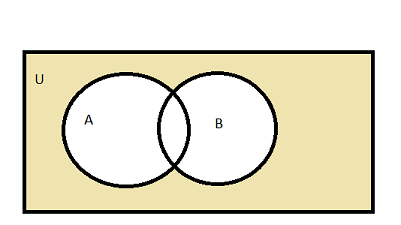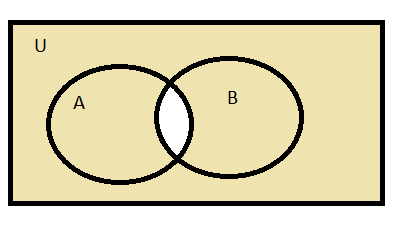Related Articles
Class 11 NCERT Solutions – Chapter 1 Sets – Exercise 1.5
• Difficulty Level : Hard
• Last Updated : 04 Dec, 2020

### (a) A’

Solution:

We know that this is the complement of set A i.e, it is the subset of U.

So, A’ = {5, 6, 7, 8, 9}

### (b) B’

Solution:

We know that this is the complement of set B i.e, it is the subset of U.

So, B’ = {1, 3, 5, 7, 9}

### (c) (A ∪ C)’

Solution:

This is the complement of union of set A and set C i.e, U – (A∪ C)

So, A∪ C = {1, 2, 3, 4, 5, 6}

=> U – (A ∪ C)

So, (A∪ C)’ = {7, 8, 9}

### (d) (A ∪ B)’

Solution:

This is complement of union of set A and set B i.e, U- (A∪B)

So, A∪ B = {1, 2, 3, 4, 6, 8}

=> U – (A ∪ B)

So, (A ∪ B)’ = {5, 7, 9}

### (e) (A’)’

Solution:

This is the complement of set A i.e, (A’)’ = A

So, (A’)’ = {1, 2, 3, 4}

### (f) (B – C)’

Solution:

(B – C) = elements in B but not in C

(B – C) = {2, 8}

=> U – (B – C)

So, (B – C)’ = {1, 3, 4, 5, 6, 7, 9}

### (a) A = {a, b, c}

Solution:

Complement of set A = A’

A’ = U – A

A’ = {a, b, c, d, e, f, g, h} – {a, b, c}

A’ = {d, e, f, g, h}

### (b) B = {d, e, f, g}

Solution:

Complement of set B = B’

B’ = U – B

B’ = {a, b, c, d, e, f, g, h} – {d, e, f, g}

B’ = {a, b, c, h}

### (c) C = {a, c, e, g}

Solution:

Complement of set C = C’

C’ = U – C

C’ = {a, b, c, d, e, f, g, h} – {a, c, e, g}

C’ = {b, d, f, h}

### (d) D = {f, g, h, a}

Solution:

Complement of set D = D’

D’ = U – D

D’ = {a, b, c, d, e, f, g, h} – {f, g, h, a}

D’ = {b, c, d, e}

### Question 3. Taking the set of natural numbers as the universal set, write down the complements of the following sets:

U = N: set of natural numbers

(a) {x : x is an even natural number}

=> {x : x is an odd natural number}

(b) {x : x is an odd natural number}

=>{x : x is an even natural number}

(c) {x : x is a positive multiple of 3}

=>{x : x∈N and x is not a multiple of 3}

(d) {x : x is a prime number}

=> {x : x is a positive composite number and x=1}

(e) {x : x is a natural number divisible by 3 and 5}

=> {x : x is a natural number that is not divisble by 3 or 5}

(f) {x : x is a perfect square}

=> {x : x∈N and x is not perfect square}

(g) {x : x is a perfect cube}

=> {x : x∈N and x is not perfect cube}

(h) {x : x + 5 = 8}

=> {x : x∈N  and x≠3}

(i) {x : 2x + 5 = 9}

=> {x : x∈N  and x≠2}

(j) {x : x ≥ 7}

=> {x : x∈N  and x<7}

(k) {x : x ∈ N and 2x + 1 > 10}

=> {x : x∈N and x≤ 9/2}

### Question 4. If U = {1, 2, 3, 4, 5, 6, 7, 8, 9}, A = {2, 4, 6, 8} and B = { 2, 3, 5, 7}. Verify that

(a) (A ∪ B)’= A’ ∩ B’

Solution:

=> (A ∪ B)’= U – (A∪B)

=> {1, 2, 3, 4, 5, 6, 7, 8, 9} – {2, 3, 4, 5, 6, 7, 8}

=> (A∪B)’ = {1, 9}

A’ ∩ B’ = (U – A) ∩ (U – B)

=> {1, 3, 5, 7, 9} ∩ {1, 4, 6, 8, 9}

=> A’ ∩ B’ = {1, 9}

Hence, Verified!!!  (A∪ B)’ = A’ ∩ B’

(b) (A ∩ B)′ = A′ ∪ B′

=> (A ∩ B)’ = U – (A ∩ B)

=> {1, 2, 3, 4, 5, 6, 7, 8, 9} – {2}

=> (A ∩ B)′ = {1, 3, 4, 5, 6, 7, 8, 9}

A’ ∪ B’= (U – A) ∪ (U – B)

=> {1, 3, 5, 7, 9} ∪ {1, 4, 6, 8, 9}

=> A′ ∪ B′ = {1, 3, 4, 5, 6, 7, 8, 9}

Hence, Verified!!!  (A ∩ B)′ = A′ ∪ B′

### Question 5. Draw appropriate Venn diagram for each of the following:

(a) (A ∪ B)′=(b) A’ ∩ B’(c) (A ∩ B)′(d) A′ ∪ B′### Question 6. Let U be the set of all triangles in a plane. If A is the set of all triangles with atleast one angle different from 60°, what is A′?

Solution:

U = set of all triangles in plane

A = set of all triangles with at least one angle different from 60°

A’ = set of all triangles with no angle different from 60° i.e, set of all triangles with all angles 60°

A’ is the set of all equilateral triangle.

### Question 7. Fill in the blanks to make each of the following a true statement :

Solution:

(a) A ∪ A′ = U

(b) ∅′ ∩ A = A

(c) A ∩ A′ = ∅

(d) U′ ∩ A =.∅

My Personal Notes arrow_drop_up